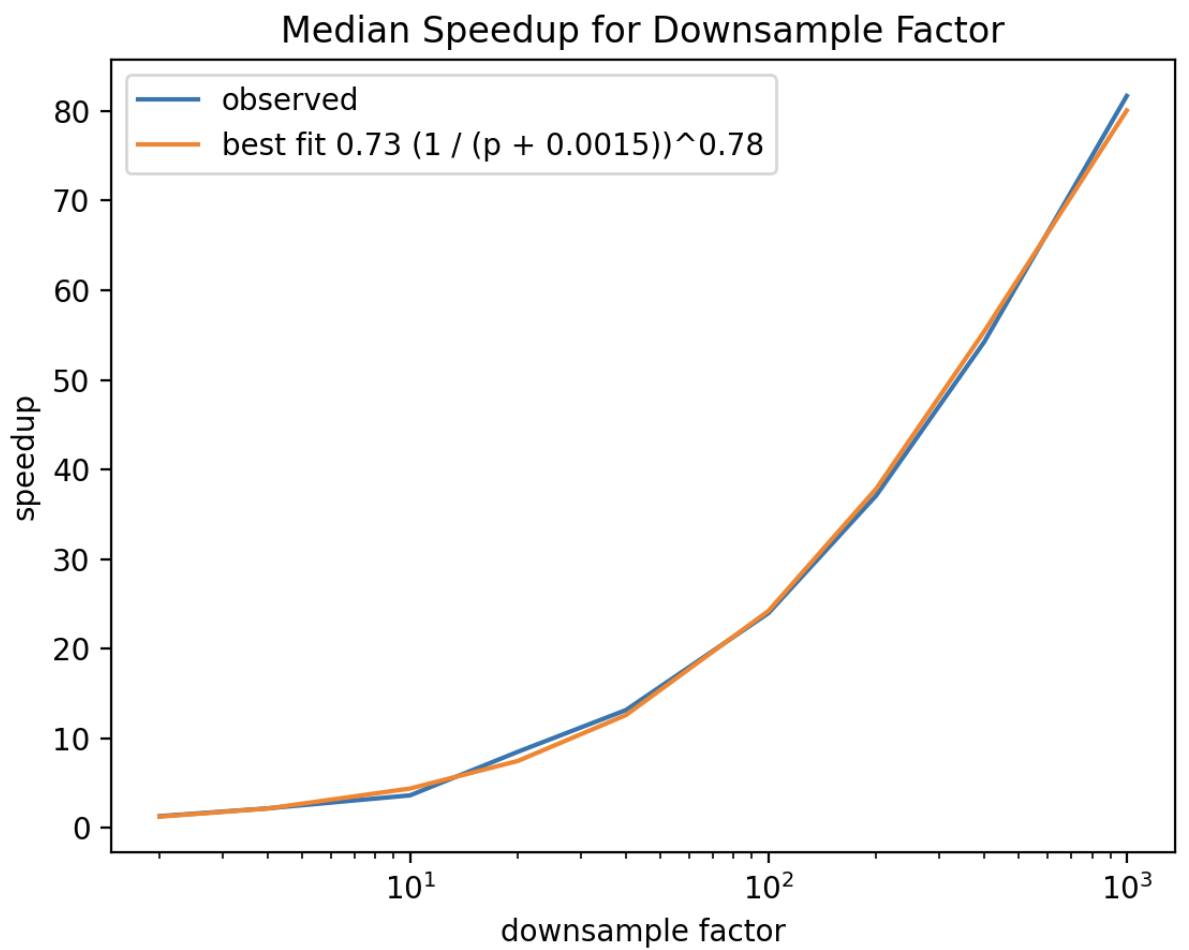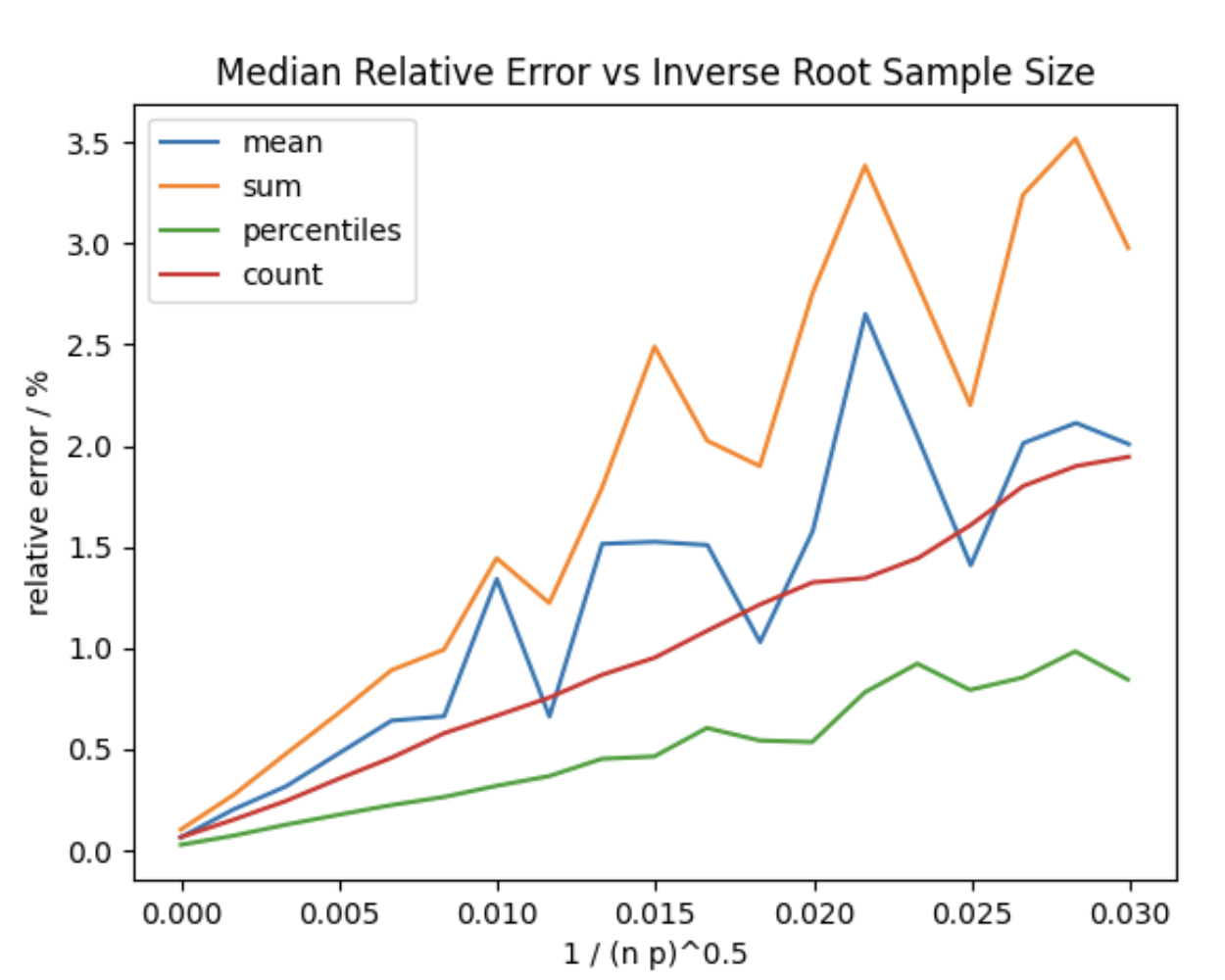## Random sampler aggregationedit

This functionality is in technical preview and may be changed or removed in a future release. Elastic will work to fix any issues, but features in technical preview are not subject to the support SLA of official GA features.

The `random_sampler` aggregation is a single bucket aggregation that randomly includes documents in the aggregated results. Sampling provides significant speed improvement at the cost of accuracy.

The sampling is accomplished by providing a random subset of the entire set of documents in a shard. If a filter query is provided in the search request, that filter is applied over the sampled subset. Consequently, if a filter is restrictive, very few documents might match; therefore, the statistics might not be as accurate.

This aggregation is not to be confused with the sampler aggregation. The sampler aggregation is not over all documents; rather, it samples the first `n` documents matched by the query.

```response = client.search(
index: 'kibana_sample_data_ecommerce',
size: 0,
track_total_hits: false,
body: {
aggregations: {
sampling: {
random_sampler: {
probability: 0.1
},
aggregations: {
price_percentiles: {
percentiles: {
field: 'taxful_total_price'
}
}
}
}
}
}
)
puts response```
```GET kibana_sample_data_ecommerce/_search?size=0&track_total_hits=false
{
"aggregations": {
"sampling": {
"random_sampler": {
"probability": 0.1
},
"aggs": {
"price_percentiles": {
"percentiles": {
"field": "taxful_total_price"
}
}
}
}
}
}```

### Top-level parameters for random_sampleredit

`probability`
(Required, float) The probability that a document will be included in the aggregated data. Must be greater than 0, less than `0.5`, or exactly `1`. The lower the probability, the fewer documents are matched.
`seed`
(Optional, integer) The seed to generate the random sampling of documents. When a seed is provided, the random subset of documents is the same between calls.

### How does the sampling work?edit

The aggregation is a random sample of all the documents in the index. In other words, the sampling is over the background set of documents. If a query is provided, a document is returned if it is matched by the query and if the document is in the random sampling. The sampling is not done over the matched documents.

Consider the set of documents `[1, 2, 3, 4, 5]`. Your query matches `[1, 3, 5]` and the randomly sampled set is `[2, 4, 5]`. In this case, the document returned would be ``.

This type of sampling provides almost linear improvement in query latency in relation to the amount by which sampling reduces the document set size:This graph is typical of the speed up for the majority of aggregations for a test data set of 63 million documents. The exact constants will depend on the data set size and the number of shards, but the form of the relationship between speed up and probability holds widely. For certain aggregations, the speed up may not be as dramatic. These aggregations have some constant overhead unrelated to the number of documents seen. Even for those aggregations, the speed improvements can be significant.

The sample set is generated by skipping documents using a geometric distribution (`(1-p)^(k-1)*p`) with success probability being the provided `probability` (`p` in the distribution equation). The values returned from the distribution indicate how many documents to skip in the background. This is equivalent to selecting documents uniformly at random. It follows that the expected number of failures before a success is `(1-p)/p`. For example, with the `"probability": 0.01`, the expected number of failures (or average number of documents skipped) would be `99` with a variance of `9900`. Consequently, if you had only 80 documents in your index or matched by your filter, you would most likely receive no results.In the above image `p` is the probability provided to the aggregation, and `n` is the number of documents matched by whatever query is provided. You can see the impact of outliers on `sum` and `mean`, but when many documents are still matched at higher sampling rates, the relative error is still low.

This represents the result of aggregations against a typical positively skewed APM data set which also has outliers in the upper tail. The linear dependence of the relative error on the sample size is found to hold widely, but the slope depends on the variation in the quantity being aggregated. As such, the variance in your own data may cause relative error rates to increase or decrease at a different rate.

### Random sampling special casesedit

All counts returned by the random sampler aggregation are scaled to ease visualizations and calculations. For example, when randomly sampling a date histogram aggregation every `doc_count` value for every bucket is scaled by the inverse of the random_sampler `probability` value. So, if `doc_count` for a bucket is `10,000` with `probability: 0.1`, the actual number of documents aggregated is `1,000`.

An exception to this is cardinality aggregation. Unique item counts are not suitable for automatic scaling. When interpreting the cardinality count, compare it to the number of sampled docs provided in the top level `doc_count` within the random_sampler aggregation. It gives you an idea of unique values as a percentage of total values. It may not reflect, however, the exact number of unique values for the given field.